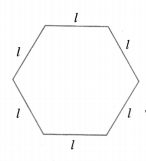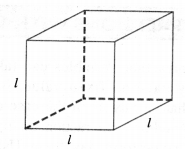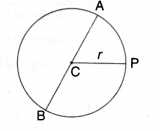NCERT Solutions for Class 6 Maths Chapter 11 Algebra Ex 11.2 are part of NCERT Solutions for Class 6 Maths. Here we have given NCERT Solutions for Class 6 Maths Chapter 11 Algebra Ex 11.2.

 Board CBSE Textbook NCERT Class Class 6 Subject Maths Chapter Chapter 11 Chapter Name Algebra Exercise Ex 11.2 Number of Questions Solved 5 Category NCERT Solutions

## NCERT Solutions for Class 6 Maths Chapter 11 Algebra Ex 11.2

Question 1.
The side of an equilateral triangle is shown by l. Express the perimeter of the equilateral triangle using l.
Solution.
Perimeter (p) of the equilateral triangle with side l = Sum of the lengths of sides of the equilateral triangle = 1 + 1 + 1 = 31.

Question 2.
The side of a regular hexagon (Fig.) is denoted by l. Express the perimeter of the hexagon using l. (Hint: A regular hexagon has all its six sides equal in length.)Solution.
Perimeter (p) of the regular hexagon with side
= Sum of the lengths of all sides of the regular hexagon
=l+l+l+l+l+l
= 6 l.

Question 3.
A cube is a three-dimensional figure as shown in Fig. It has six faces and all of them are identical squares. The length of an edge of the cube is given by l. Find the formula for the total length of the
edges of a cube.Solution.
Total length (L) of the edges of a cube
= Sum of the lengths of all (12) edges of the cube
= l+l+l+l+l+l+l+l+l+l+l+l
=12 l

Question 4.
The diameter of a circle is a line which joins two points on the circle and also passes through the center of the circle. (In the adjoining figure (Fig.) AB is a diameter of the circle; C is its center.) Express the diameter of the circle (d) in terms of its radius (r).Solution.
AB = AC + CB
⇒ d=r+r ⇒ d=2r

Question 5.
To find the sum of three numbers 14, 27 and 13, we can have two ways.
(a) We may first add 14 and 27 to get 41 and then add 13 to it to get the total sum 54 or
(b) We may add 27 and 13 to get 40 and then add 14 to get the sum 54. Thus,
(14 + 27) + 13 = 14 + (27 + 13)
This can be done for any three numbers. This property is known as the associativity of the addition of
numbers. Express this property which we have already studied in the chapter on Whole Numbers, in a general way, by using variables a, b and c.
Solution.
Let a, b and c be three variables, each of which can take any numerical value. Then.
(a + b) + c = a + (b + c)
This property is known as the associativity of the addition of numbers.

We hope the NCERT Solutions for Class 6 Maths Chapter 11 Algebra Ex 11.2 help you. If you have any query regarding. NCERT Solutions for Class 6 Maths Chapter 11 Algebra Ex 11.2, drop a comment below and we will get back to you at the earliest.